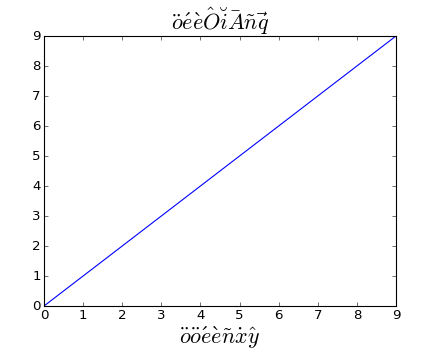# pylab_examples example code: accented_text.py¶#!/usr/bin/env python
"""
matplotlib supports accented characters via TeX mathtext

The following accents are provided: \hat, \breve, \grave, \bar,
\acute, \tilde, \vec, \dot, \ddot.  All of them have the same syntax,
eg to make an overbar you do \bar{o} or to make an o umlaut you do
\ddot{o}.  The shortcuts are also provided, eg: \"o \'e \e \~n \.x
\^y

"""
from pylab import *

axes([0.1, 0.15, 0.8, 0.75])
plot(range(10))

title(r'$\ddot{o}\acute{e}\grave{e}\hat{O}\breve{i}\bar{A}\tilde{n}\vec{q}$', fontsize=20)
# shorthand is also supported and curly's are optional
xlabel(r"""$\"o\ddot o \'e\e\~n\.x\^y$""", fontsize=20)

show()


Keywords: python, matplotlib, pylab, example, codex (see Search examples)